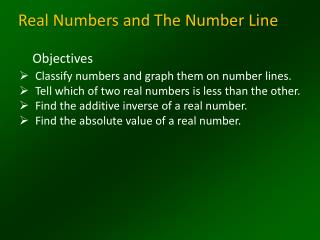DownloadDownload PresentationReal Numbers and The Number Line

Real Numbers and The Number Line

Télécharger la présentationReal Numbers and The Number Line

- - - - - - - - - - - - - - - - - - - - - - - - - - - E N D - - - - - - - - - - - - - - - - - - - - - - - - - - -
Presentation Transcript

1. Real Numbers and The Number Line Objectives • Classify numbers and graph them on number lines. • Tell which of two real numbers is less than the other. • Find the additive inverse of a real number. • Find the absolute value of a real number.

2. Square Root A square root is one of two equal factors of a number. For example one square root of 64 is 8 since A number like 64, whose square root is a rational number is called a perfect square Example

3. Square Root The symbol called a radical sign, is used to indicate the principal square root of the expression under the radical sign For example Note that is not the same as . is not a real number since no real number multiplied by itself is negative

4. Find Square Roots

5. Objective 1 Classify numbers and graph them on number lines NATURAL NUMBERS {1, 2, 3,…} WHOLE NUMBERS {0, 1, 2, 3,…} INTEGERS {…-2, -1, 0, 1, 2,…} RATIONAL NUMBERS Any number that can be written in fraction form is a rational number. This includes integers, terminating decimals, and repeating decimals. Irrational numbers Numbers that can not be expressed as rational numbers.

6. Real Numbers Rational Numbers Integers Irrational Numbers Whole Numbers Natural Numbers The set of Real Numbers consists of the set of rational numbers and the set of irrational numbers.

7. Examples of Rational numbers 5 0.3 - 7 0

8. EXAMPLES OF IRRATIONAL NUMBERS the is a whole number, but the , since It lies between 5 and 6,must be irrational.

9. Name the sets of numbers to which belongs. Name the sets of numbers to which belongs. lies between 2 and 3 so it is not a whole number. Example 2-1c Answer:irrational and real Answer:natural , whole , integer , rational, and real

10. Name the sets of numbers to which each number belongs. a. b. c. d. Example 2-1f Answer:rational and real Answer:rational and real Answer:irrational and real Answer:natural , whole , integer rational and real (R)

12. Completeness Property Each point on the number line corresponds to exactly one real number Example: Graph: ( -4, -2, 0, ,4, )

13. Objective 2 Tell which of two real numbers is less than the other Since the numbers are equal. Answer: Example 7-4a Replace the with <, >, or = to make the sentence true.

14. Objective 2 Tell which of two real numbers is less than the other Answer: Example 7-4b Replace the with <, >, or = to make the sentence true.

15. Objective 2 Order Real numbers from least to greatest

16. Order Real numbers from least to greatest From the least to the greatest

17. Objective 3 Find the additive inverse of a real number. The opposite of 5 is The opposite of 5 is The opposite of -3 is When you add two opposite numbers , the sum is always 0.

18. Objective 4 Find the absolute value of a real number The absolute value of any number n is its distance from zero on a number line and is represented as Example: and 3 units 3 units

19. END OF LESSON